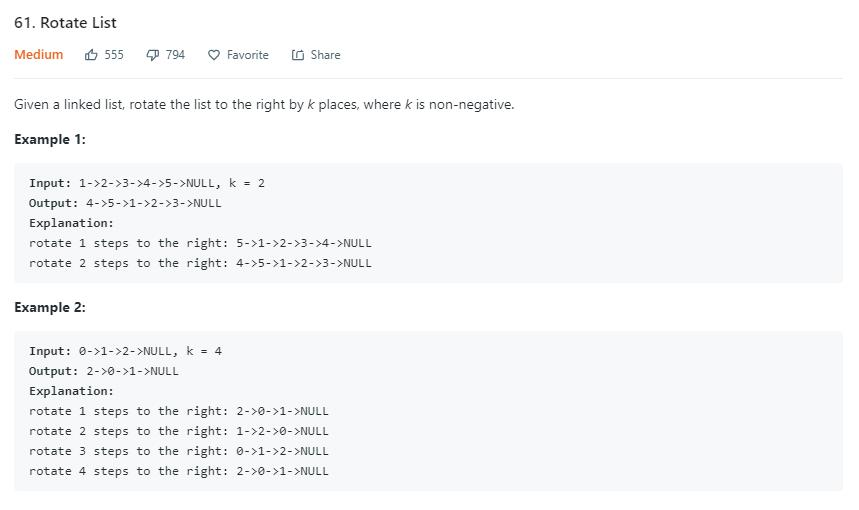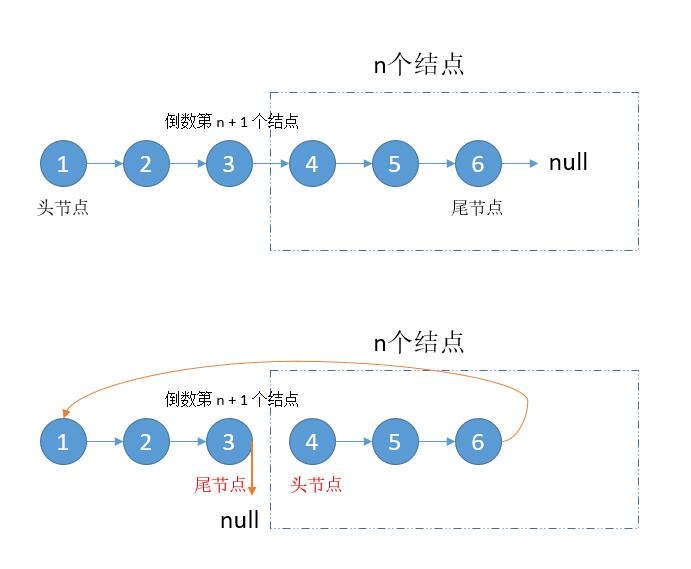# 题目描述（中等难度）# 解法一public ListNode rotateRight(ListNode head, int k) {
if (head == null || k == 0) {
}
int len = 0;
ListNode tail = null;
//求出链表长度，保存尾指针
while (h != null) {
h = h.next;
len++;
if (h != null) {
tail = h;
}
}
//求出需要整体移动多少个节点
int n = k % len;
if (n == 0) {
}

//利用快慢指针找出倒数 n + 1 个节点的指针，用 slow 保存
while (n >= 0) {
fast = fast.next;
n--;
}
while (fast != null) {
slow = slow.next;
fast = fast.next;
}
//尾指针指向头结点
//头指针更新为倒数第 n 个节点
//尾指针置为 null
slow.next = null;
}


ListNode slow = head;
for (int i = 1; i < len - n; i++) {
slow = slow.next;
}